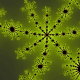Welcome to AWESOME ALGEBRA!

Pearson Maths 7 Chapter 5:

Textbook 5.1-5.6

Textbook 5.7 – 5.8

Algebraic concepts in this unit:

5.1 Pronumerals and variables

What number am I?

Sometimes, Always or Never True

5.2 Terms, expressions and equations

Maths tricks

Creating expressions

5.3 Using rules

5.4 Formulas and substitution

Substitution practice

Substitution (challenging)

Substitution (2 variables)

Substitution with fractions

5.5 Patterns and rules

Practice finding patterns and rules

Finding rules (functions)

Leaping frogs

5.6 Problem solving using algebra

5.7 Simplifying expressions with addition and subtraction

5. 8 Patterns and plotting points

5.9 Interpreting graphs

Some interesting algebra clips:

A little history of Maths

What is algebra, really?

Why learn algebra?